#Quality Digest

Featured Product
This Week in Quality Digest Live
Statistics Features
Donald J. Wheeler
What do predictable processes have in common with chaos?
William A. Levinson
People can draw the wrong conclusions due to survivor, survey, and bad news bias.
Donald J. Wheeler
Does your approach do what you need?
Paul Laughlin
Correlation vs. causality
Donald J. Wheeler
In spite of what everyone says to the contrary
Statistics News
New capability delivers deeper productivity insights to help manufacturers meet labor challenges
Day and a half workshop to learn, retain, and transfer GD&T knowledge across an organization
Elsmar Cove is a leading forum for quality and standards compliance
InfinityQS’ quality solutions have helped cold food and beverage manufacturers around the world optimize quality and safety
User friendly graphical user interface makes the R-based statistical engine easily accessible to anyone
Collect measurements, visual defect information, simple Go/No-Go situations from any online device
Good quality is adding an average of 11 percent to organizations’ revenue growth
Ability to subscribe with single-user minimum, floating license, and no long-term commitment
A guide for practitioners and managersBio

Statistics

## Avoiding Bias Correction Confusion

### When should we use the various bias correction factors?

Published: Monday, August 5, 2019 - 12:03

Recently I have had several questions about which bias correction factors to use when working with industrial data. Some books use one formula, other books use another, and the software may use a third formula. Which one is right? This article will help you find an answer.

Before we can meaningfully discuss different bias correction factors we need to understand what they do. To this end we must make a distinction between parameters for a probability model and statistics computed from the data. So we shall go back to the origin of our data and move forward.

A statistic is simply a function of the data. Data plus arithmetic equals a statistic. Since arithmetic cannot create meaning, it is the context for the data that gives specific meaning to any statistic. Thus, we will have to begin with the progression from a physical process to a probability model, and then we can look at how the notion of a probability model frames the way we use our statistics.

Assume that we have a process that is producing some product, and assume that periodic checks are made upon some product characteristic. These checks will result in a sequence of values that could be written as: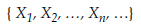When we compute descriptive statistics from the first n values of this sequence there are two fundamental questions of interest: “How well do these statistics characterize the product produced during the time period covered?” and “Can we use these statistics to predict what the process will produce in the future?” Everything we do in practice hangs on these two questions, and the answers to these questions require an extrapolation. We have to extrapolate from the product we have measured to the product not measured. And this applies both to product produced in the past and product to be produced in the future. Therefore, we have to know when such extrapolations make sense.

Walter Shewhart provided a succinct answer to the question of extrapolation. Paraphrasing, he said: A process will be predictable when, through the use of past experience, we can describe, at least within limits, how the process will behave in the future. If the process has displayed predictable operation in the past, and if there is no evidence of unpredictable operation in the present, then the extrapolation from the data to the underlying process will be credible. Moreover, as long as the process continues to be operated predictably, the statistics based upon the historical data will continue to characterize the production process and the process outcomes.

However, when the process shows evidence of unpredictable operation, we are no longer justified in extrapolating from the data to the process. With the strong evidence that the process is changing that is provided by a process behavior chart, any attempt to use the historical data to predict the future can only be based on wishful thinking.

### From a process to the notion of a distribution

When a production process is operated predictably it will be characterized by data that are homogeneous—measurements that display a consistent and recurring pattern of variation. This will result in a histogram that will essentially look more or less the same from time period to time period. This stable pattern of variation might then be approximated by some continuous probability model, f(x). This conceptual probability model, f(x), will be a mathematical function that can be characterized by parameters such as the center of mass, MEAN(X), and the radius of gyration (also known as the standard deviation parameter) SD(X). (In actual practice we neither need to draw the series of histograms, nor choose a probability model, but with a predictable process such actions would make sense.)Figure 1: When a probability model makes sense

However, in practice, we will never have enough data to ever fully define a specific probability model in the manner implied by figure 1. So even though we may have a predictable process, we will not be able to directly compute our process parameters. Fortunately we can still characterize our process using estimates of the process parameters based on the statistics computed from the process data. Before we look at how this is done we need to consider what happens when a process is operated unpredictably.

### When the notion of process parameters evaporates

When a process displays unplanned and unexpected changes it is said to be operated unpredictably. As a result the data will not be homogeneous and the histograms will be changing from time period to time period. So while we can always calculate our summary statistics, and while these summary statistics might somehow describe the past data, the idea that we can find a single probability model that will characterize the process outcomes no longer makes sense.Figure 2: When a probability model does not make sense

When we cannot use a single probability model to describe the process outcomes the notion of process parameters will evaporate. Here we can no longer meaningfully talk about a process mean, a process variance, or a process standard deviation parameter. While we may still compute our various statistics, and while may still use these statistics to characterize the process behavior as being either predictable or unpredictable, the statistics themselves will no longer represent specific process parameters. Our statistics only become meaningful estimators of process parameters when the process is being operated predictably. This is why is it crucial to make a distinction between statistics, which are functions of the data, and parameters, which are descriptive constants for a predictable process. All that follows will only make sense when we are working with a predictable process.

### Estimators for dispersion parameters

When we have a reasonably predictable process we generally want to characterize our process location and dispersion.  The average statistic provides an intuitive estimator for the process mean, MEAN(X).  The complexity begins when we seek to characterize dispersion.  First we have to decide if we need to estimate the process standard deviation, SD(X), or the process variance, VAR(X).  Next we have to decide which dispersion statistic we shall use.  While there are many possible choices here, the three most commonly used are the standard deviation statistic, s, the variance statistic, s2, and the range statistic, R.  Finally we have to choose whether to use a biased estimator or an unbiased estimator.  Thus, we have a matrix of dispersion estimators as shown in figure 3.  The three quantities shown in the denominators are known as bias correction factors. A table of these factors is given at the end of this paper.Figure 3: Choices when estimating dispersion

So how do we choose between these various formulas?  In most cases the formula is already incorporated into the technique, so you do not have to choose.  But when given a choice the unbiased estimators are generally preferred.

### Unbiased estimators

An estimator of a parameter is said to be unbiased when it is, on the average, neither too large nor too small.

For example, in figure 3, the variance statistic is an unbiased estimator for VAR(X) because the mean of the distribution of the variance statistic is equal to the parameter value.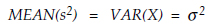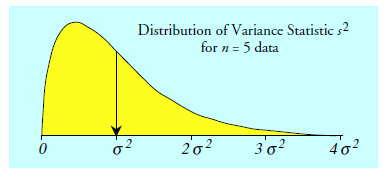Figure 4: The distribution of the variance statistic when n = 5

Thus, the property of being unbiased is a property of the distribution of the formula for the statistic (i.e., a random variable) rather than being a property of the computed value (an observation on the random variable).  An observed value for the variance statistic might fall anywhere under the curve in figure 4, but the mean value of all possible observations of the variance statistic will be equal to the value of VAR(X).  Thus, we may write:### Biased estimators

When we take the square root of the variance statistic we end up with the standard deviation statistic, s, which we often use as an estimator for the SD(X) parameter. Figure 5 shows the distribution for this estimator.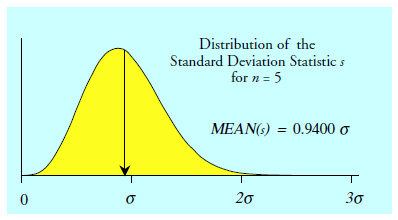Figure 5: The distribution of the standard deviation statistic when n = 5

The mean of the distribution in figure 5 is only equal to 0.9400 times SD(X).  This means that, when n = 5, the standard deviation statistic will turn out to be about 6 percent too small on the average, and so it is said to be a biased estimator for SD(X)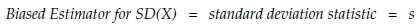This bias is a consequence of the square root transformation.  The property of being unbiased is only preserved by linear transformations (such as averaging) and it is lost whenever we perform a non-linear transformation (such as squaring or taking a square root).  So while the variance statistic is an unbiased estimator for VAR(X), the standard deviation statistic is a biased estimator for SD(X), and this property is inherent in the definition of an unbiased estimator.  The square of an unbiased estimator will always be biased, and the square root of an unbiased estimator will always be biased.

### Removing the bias

Whenever the mean value for the distribution of a statistic is some multiple of the value of a parameter, the statistic may be converted into an unbiased estimator for that parameter by the use of a bias correction factor. Figure 5 suggests that, for n = 5: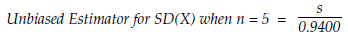The usual symbol for these bias correction factors for the standard deviation statistic is a lower-case c with a subscript of 4: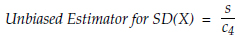The distribution of the range statistic for subgroups of size n = 5 is shown in figure 6.  On the average, subgroups of size five will have a range statistic that is 2.326 times the SD(X) parameter.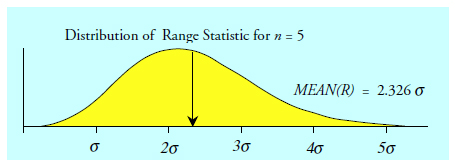Figure 6: The distribution of the range statistic when n = 5

Hence the bias correction factor for the range of five data is 2.326.  Collectively we denote the bias correction factors for the range statistic using a lower-case d with a subscript of 2: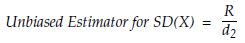Figure 7 shows the bias-adjusted distributions of both the standard deviation statistic and the range statistic for n = 5.  There is no practical difference between these unbiased estimators of SD(X).  They both provide essentially the same information with equal precision.  This effective equivalence holds for subgroup sizes up to n = 10.Figure 7: Bias-adjusted distributions when n = 5

Since the property of being unbiased is only preserved by linear transformations, we know that the square of either of the unbiased estimators for SD(X) given above will result in a biased estimator for VAR(X).  Specifically, the square of our bias-adjusted range will result in a biased estimator for VAR(X).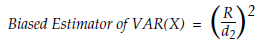### An unbiased estimator for variance based on the range

In 1950, another bias correction factor was defined which allowed a range or an average range to be used as an unbiased estimator for VAR(X):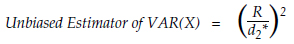The following will illustrate how and why this correction factor differs from the one for estimating SD(X).  Figure 8 shows the distribution for the range statistic for one subgroup of size 2. There we see that the bias correction factor for estimating SD(X) when n = 2 is: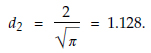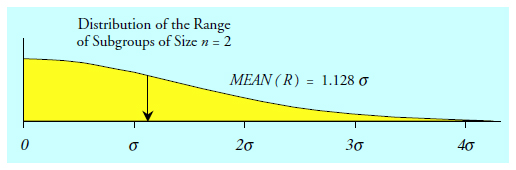Figure 8: Distribution of range statistic when n = 2

When we square the range statistic in figure 8 we end up with the transformed version of the chi-square distribution with one degree of freedom shown in figure 9.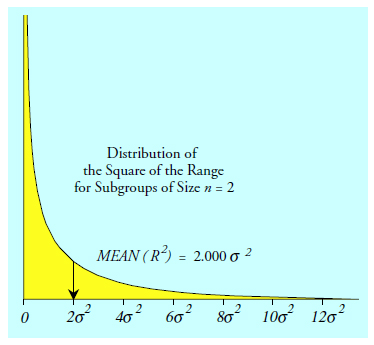Figure 9: Distribution of range statistic squared when n = 2

When n = 2, to obtain an unbiased estimator for VAR(X) based on the square of the range we will need to divide the squared range by 2.000.  Alternatively, we could divide the range itself by the square root of 2 and then square the result.  Thus, for n = 2 and k = 1: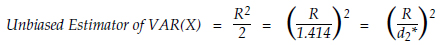### Biased correction for average dispersion statistics

Figure 3 and all of the preceding discussion was focused on what happens with dispersion statistics computed from one group of n data.  Here we will address what happens when we are working with k subgroups of size n and use an average dispersion statistic.

Figure 10 lists the estimators based on the average standard deviation statistic, the pooled variance statistic, and the average range statistic.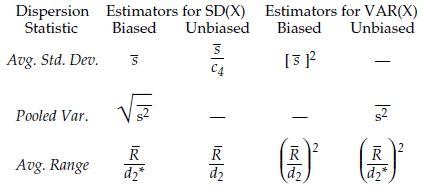Figure 10: Estimating dispersion using k subgroups of size n

When we average k dispersion statistics, each of which is based on the same amount of data, we can simply use the bias correction factor that is appropriate for a single one of the dispersion statistics to obtain an unbiased estimator.  Remember, linear transformations do not affect the property of being unbiased.  Thus, when we average the first three unbiased estimators from figure 3 we get the following: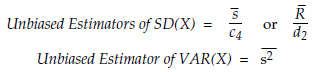For this reason we do not have to be concerned with the number of subgroups involved when averaging unbiased estimators.  This is illustrated in figure 11.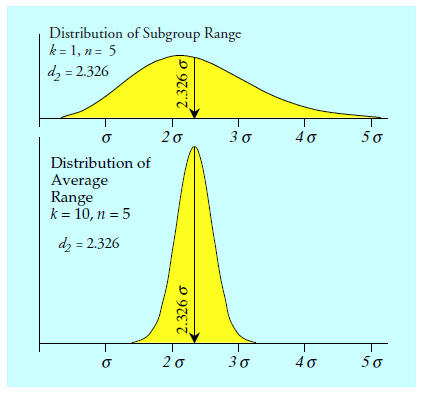Figure 11: Computing an average does not affect the mean value

However, when using the range to estimate VAR(X) things are different.  The fact that we are first going to compute the average range and then square it to obtain our unbiased estimator for VAR(X) makes the bias correction factor dependent upon both n and k.  The nonlinear transformation in the middle of the formula effectively creates this dependence.

When we square the random variables defined in figure 11 we get the two distributions shown in figure 12. Because the original distributions differ, the distributions of the squared random variables differ. Specifically, the distributions in figure 12 have different mean values. When we take the square root of these mean values we find that the appropriate bias correction factor for a range-based estimate of VAR(X) is different when n = 5 and k = 1 from what it is when n = 5 and k = 10. Thus, the bias correction factors for estimating VAR(X) will depend upon both n and k.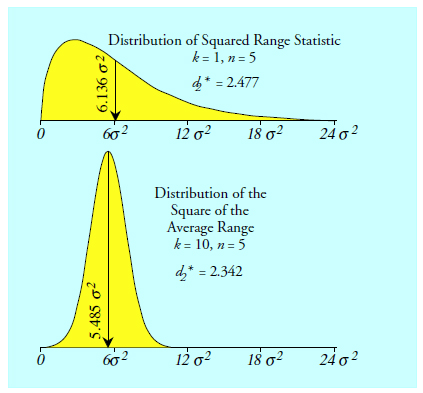Figure 12: Squaring the distributions in figure 11 affects the mean values differently

### So which bias correction factor should we use?

We prefer to use unbiased estimators simply because it always sounds better to be unbiased! However, it is important to note that in the distributions in figures 4 through 12 an estimate can fall anywhere under the curves. Your statistics are not going to give you values that fall right at the mean of these distributions. So, in practice, the numbers we compute are always biased in the sense that they are not equal to the unknown parameter value. We have to be content with the knowledge that they are merely in the right ballpark.

To illustrate this point an artificial example is used (so that we actually know the parameter values). Ten values were obtained at random from a normal probability model having a mean of 15 and a variance of 4. They were split into two subgroups of size five and the dispersion statistics were computed.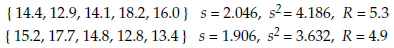The average standard deviation statistic is 1.975. The pooled variance statistic is 3.909. And the average range is 5.1. The bias correction factors are:The formulas in figure 10 result in the nine estimates listed in figure 13.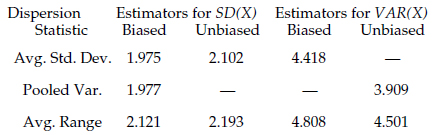Figure 13: Nine estimates of dispersion using two subgroups of size 5

The knowledge that we have used a formula for an unbiased estimator does not convey any information about the distance between our estimate and the parameter value. Unbiased estimators are not categorically closer to the parameter value than are biased estimators. They just sound better.

The estimators in figure 10 are all within-subgroup estimators. In practice, these within-subgroup estimates of dispersion are essentially interchangeable, especially if we take into account their different degrees of freedom. Using one of these estimates in place of another estimate of the same parameter will not make any real difference in your analysis.

This makes the distinction about whether to use a biased or unbiased estimator a tempest in a teapot. The difference between the various estimators will always be trivial in comparison with the uncertainty in the dispersion statistics themselves. So, while we might prefer to use unbiased estimators, this is not something to obsess about. Use the formulas given as part of the technique, and stop trying to “fine tune” things with alternative formulas.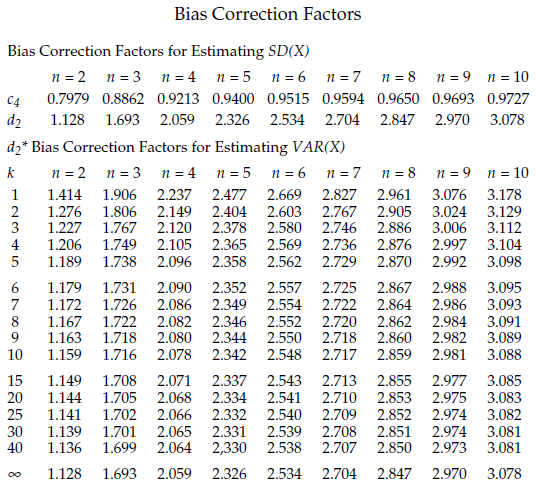### Discuss### Donald J. Wheeler

Find out about Dr. Wheeler’s virtual seminars for 2022 at www.spcpress.com. Dr. Wheeler is a fellow of both the American Statistical Association and the American Society for Quality who has taught more than 1,000 seminars in 17 countries on six continents. He welcomes your questions; you can contact him at djwheeler@spcpress.com.

### Important points!

We often say, "If there is one thing that everyone should understand..."

I remember Don stating in an Advanced Topics seminar that--shortly before he died--David Chambers told him something to the effect that if he (Chambers) had had it to do over again, he would do the ball socket/rational subgrouping exercise in every seminar they did. He thought it was that important.

The first few paragraphs of this article are something every Stats 101 student should be required to understand; they sort of describe some of the basic characteristics of what Deming called analytic studies. In most Stats 101-level courses, students are introduced to descriptive statistics, probability theory, and then distribution theory, but it's all in the context of enumerative studies, where we take samples from a (in principle) static population and extrapolate from those sample statistics to describe the population.

These concepts become the basis of everything else in college stats - a result of statistics being corralled under the school of mathematics at the beginning of the last century instead of the school of physical sciences where it belonged (I can't take credit for that; George Box wrote a paper about it, but I certainly agree with the sentiment). Tests of hypotheses and confidence intervals and most of the other concepts you learn unless you get lucky and take some industrial engineering classes taught by someone who undersstands analytic studies - all of these are based on that idea that there is a population and that we can describe some actual parameters of that population (at least for the time period of the sample).

Shewhart had another problem - the one most of us have in this game - the problem of process data, where time is an important context. Although Deming named it, Shewhart developed the idea of the analytic study, which was necessary because he was (as Don describes so well above) taking data from a dynamic stream, not a static population. In analytic studies we don't worry about populations, because they don't exist as a practical matter - you could argue that if a process is stable then there is a population of data that is developed while the process remains stable, but who cares? That would be semantic angels on the head of a pin (in my humble opinion). We are not trying to use a sample to represent and extrapolate from that sample to a population. We are trying to use subgroups to represent the past and present and extrapolate to the future.

If more authors who try to write about SPC understood this distinction, we would not have so many textbooks talking about "sample size" in control charts when they mean "subgroup size." We wouldn't have people writing that you have to test for normality (or for any other shape) before you establish that a process is in control and internally homogeneous (because only then can we assume that we have any distributional model at all to work with).

Thank you, Don, for reminding us again of this fundamental principle.